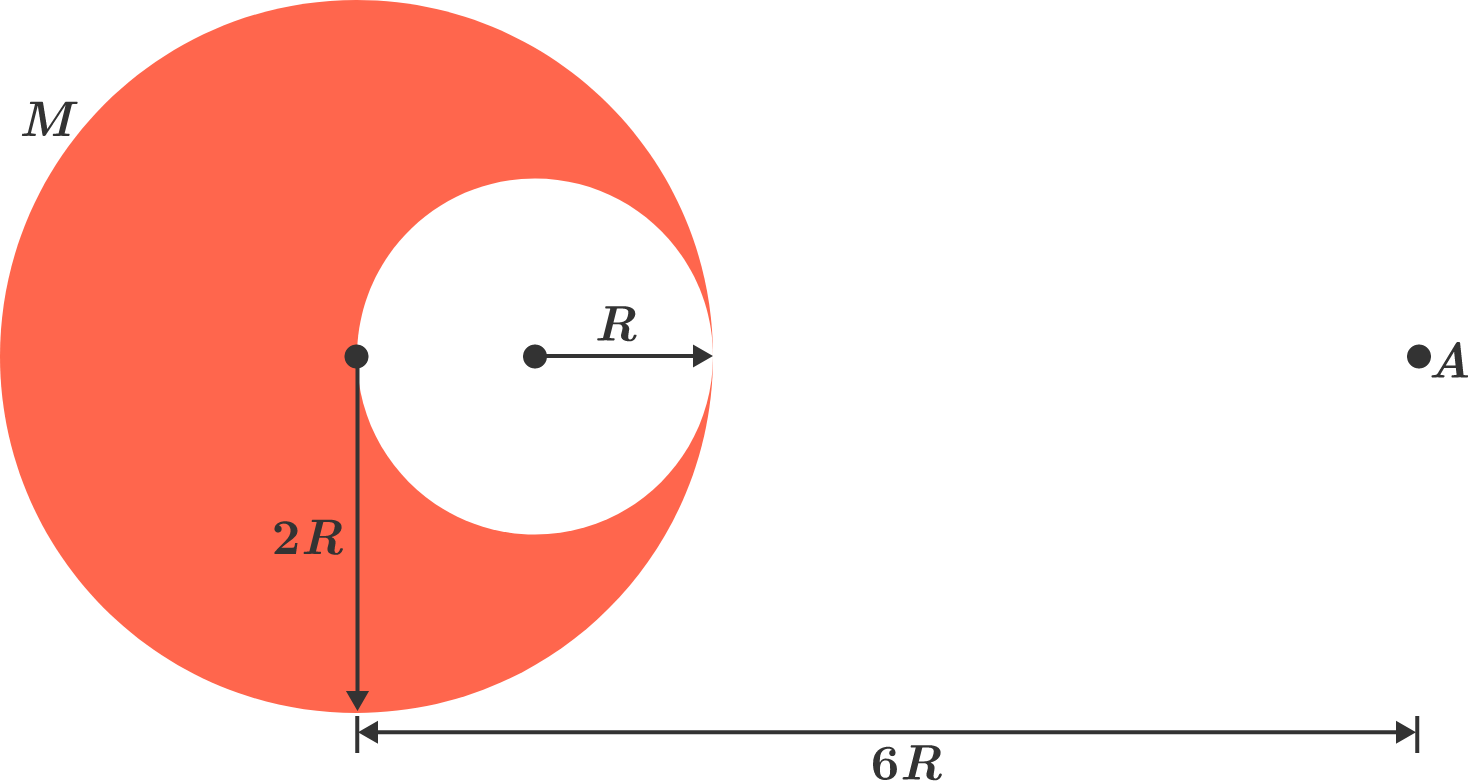# Gravitational field intensity of a carved out spherical bodyFrom a uniform sphere of radius $2R$, a spherical cavity of radius $R$ is cut in such a way that the sphere and the spherical cavity share a common tangent, as shown in the diagram. The mass of the new body is $M$. Find the gravitational field intensity at point $A$ which is at a distance of $6R$ from the center of the sphere.

If this value can be expressed as $\dfrac{a}{b} \cdot \dfrac{GM}{R^2}$, where $a$ and $b$ are coprime positive integers, then evaluate $a+b$.

Details and Assumptions:

• Point $A$ is at a distance of $6R$ from the geometrical center of the original, larger sphere, not from the center of mass of the newly formed body.
• Point $A$ lies such that it is collinear with the centers of the sphere and the spherical cavity and nearer to the common tangent shared by them.
• $G$ denotes the universal gravitational constant: $G = 6.674 \times 10^{-11} \text{ m}^3 \text{ kg}^{-1} \text{ s}^{-2}.$
• Neglect Earth's gravitational field and deformities within the sphere.
×